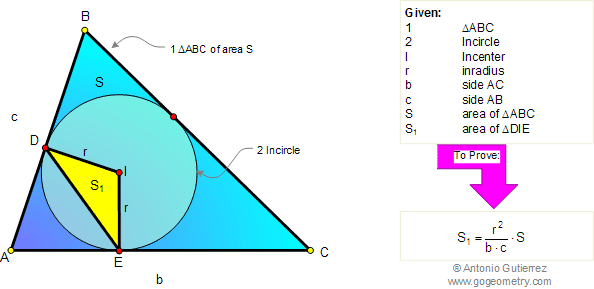# Geometry Problem 80: Triangle Area, Side, Inradius, Circumradius. High School, College, Math Education

 Given a triangle ABC of area S, the incircle of center I and inradius r. If AC = b and AB = c, prove that area of triangle DIE is $$S_1 = \dfrac{r^2}{b\cdot c}\cdot S$$.See also: Poster of problem 80
 Home | Search | Geometry | Problems | All Problems | Open Problems | Visual Index | 71-80 | Wolfram Demonstrations Project | Circle Tangent Line | Email View or post a solution